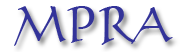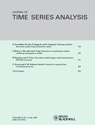Home

Bartlett's formula for a general class of non linear processes

Christian Francq and Jean-Michel Zakoïan
Keywords: Bartlett's formula, nonlinear time series model, sample autocorrelation, GARCH model, weak white noise
Abstract: A Bartlett-type formula is proposed for the asymptotic distribution of the sample autocorrelations of nonlinear processes. The asymptotic covariances between sample autocorrelations are expressed as the sum of two terms. The first term corresponds to the standard Bartlett's formula for linear processes, involving only the autocorrelation function of the observed process. The second term, which is specific to nonlinear processes, involves the autocorrelation function of the observed process, the kurtosis of the linear innovation process and the autocorrelation function of its square. This formula is obtained under a symmetry assumption on the linear innovation process. It is illustrated on ARMA-GARCH models and compared to the standard formula. An empirical application on financial time series is proposed.MPRA working paperJournal of Time Series Analysis, 30, 449-465, 2009.
Stock market indices: CAC 40, DAX, FTSE, Nikkei 40, SMI, SP500 (obtained from yahoo).
R codeused to obtain Figures 2 and 3